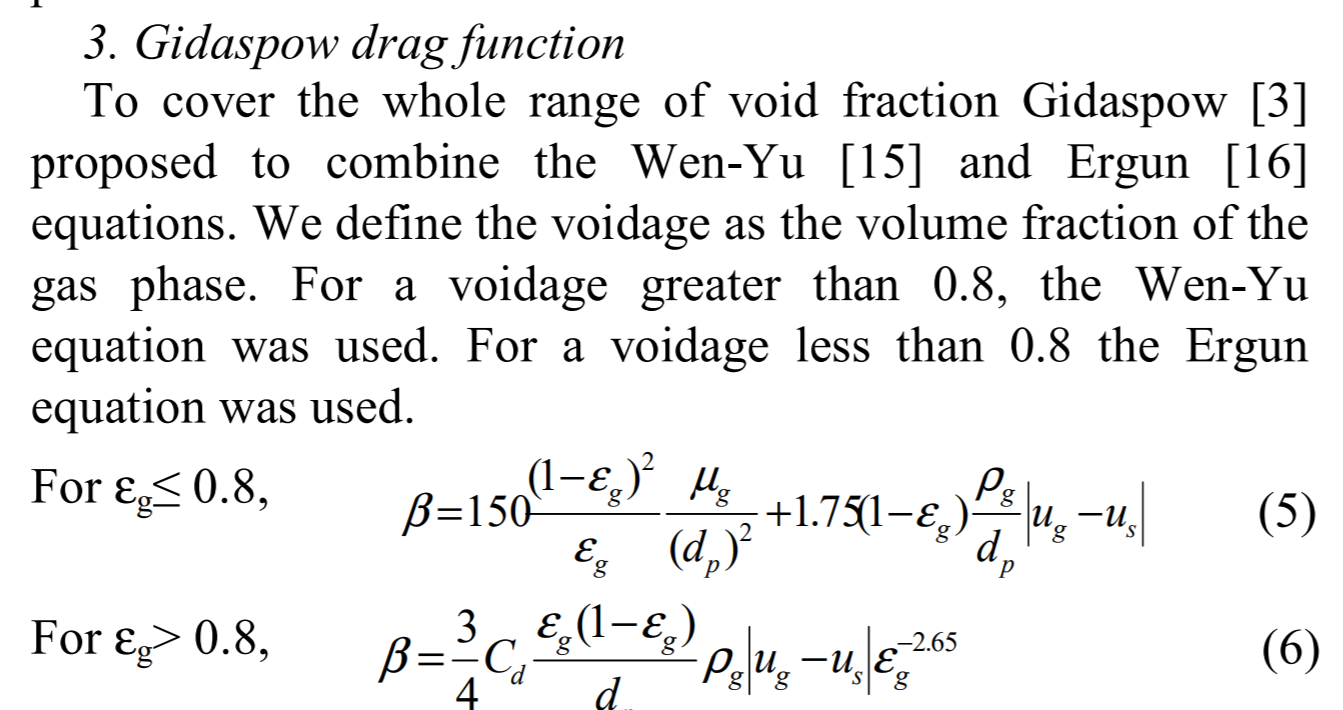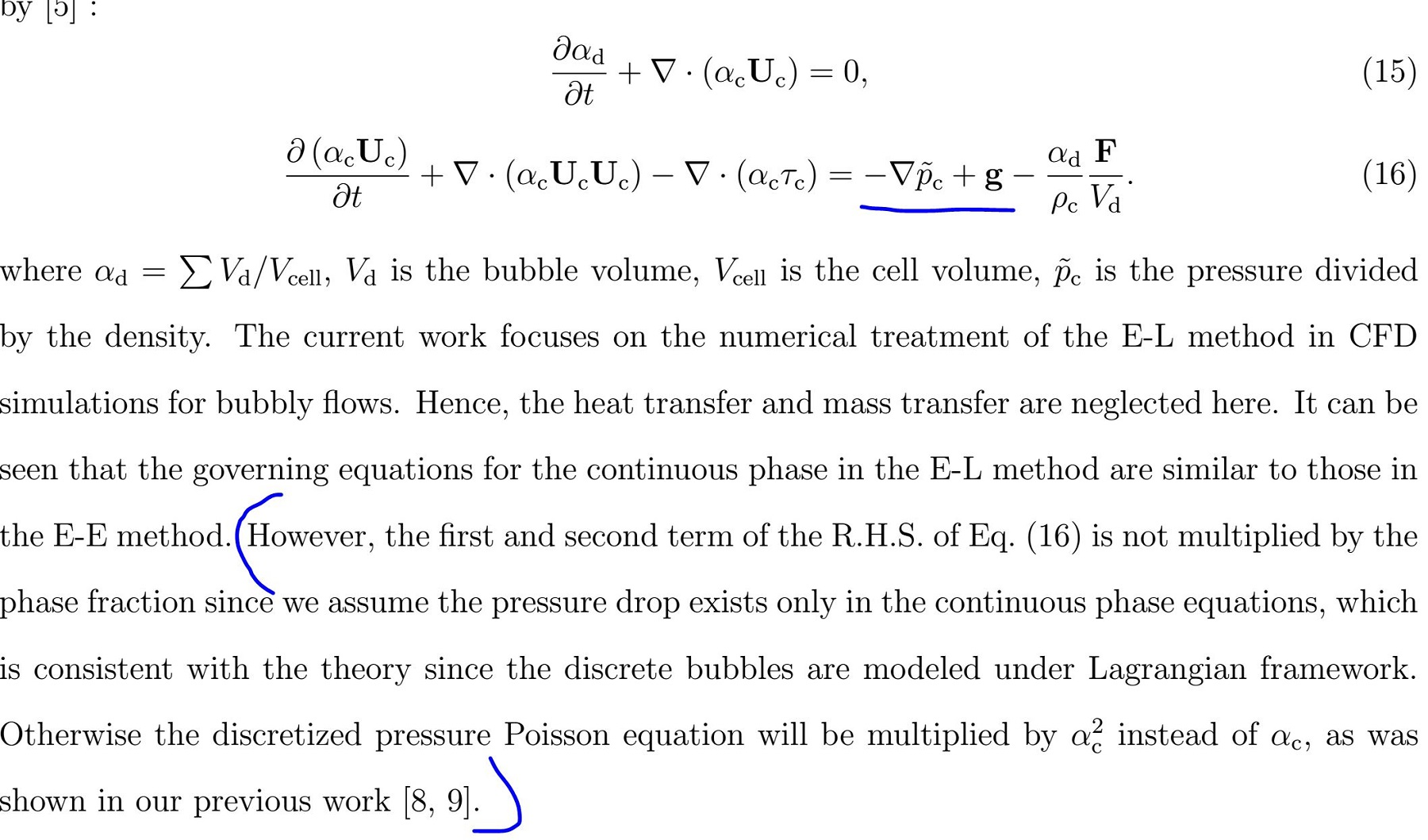# particleforce类内ErgunWenYuDrag的表达式感觉有错误

• 最近在研究DPMFoam求解器时，看到了ErgunWenYuDrag力的表达式源程序是：

src/lagrangian/intermediate/submodels/Kinematic/ParticleForces/Drag/ErgunWenYuDrag/ErgunWenYuDragForce.C +97
template<class CloudType>
Foam::forceSuSp Foam::ErgunWenYuDragForce<CloudType>::calcCoupled
(
const typename CloudType::parcelType& p,
const scalar dt,
const scalar mass,
const scalar Re,
const scalar muc
） const
{
scalar alphac(alphac_[p.cell()]);

if (alphac < 0.8)
{
return forceSuSp
(
Zero,
(mass/p.rho())
*(150.0*(1.0 - alphac)/alphac + 1.75*Re)*muc/(alphac*sqr(p.d()))//感觉和原始文献里面有很大的区别
);
}
else
{
return forceSuSp
(
Zero,
(mass/p.rho())
*0.75*CdRe(alphac*Re)*muc*pow(alphac, -2.65)/(alphac*sqr(p.d()))//这个也是
);
}
}


公式显示貌似有些问题。
翻译一下公式是:
\begin{equation}
\frac{m_{d}}{\rho _{d}}\frac{\left ( \frac{150\left ( 1-\alpha _{c} \right )}{\alpha _{c}} +1.75Re\right)\mu _{c}}{\alpha _{c}d^{2}}\left( \alpha {c}< 0.8\right )
\end{equation}
原始文献里面的计算公式:
\begin{equation}
\frac{150\left(1-\alpha{c} \right )^{2}\mu {c}}{\alpha{c}d^{2}}+1.75\frac{\rho _{c}\alpha {d}\left | v{r} \right |}{d}
\end{equation}
两个差了很多。不知道有木有大神解答一下，纠结了一整天了• 曳力这面计算公式要稍微注意一下，openfoam那面用的是CdRe，而不是Cd*Re，约掉了一些项，我没太对比，你注意下这个问题看看能否一致

• 好的，谢谢李老师，我再研究研究

• Good, 等着你的反馈• 经过仔细比对，发现OF里面ErgunWenYuDrag模型的实现确实有些许问题，下面是我的详细分析。
首先，参照文献Ding and Gidaspow, 1990所述,欧拉-欧拉模型连续相方程为：
\begin{equation}
\label{E1}
\frac{\partial \left( \alpha_{c}\rho_{c}\mathbf{U}_ {c}\right)}{\partial b}+\nabla \cdot \left( \alpha_{c}\rho_{c}\mathbf{U}_ {c}\mathbf{U}_ {c}\right )-\nabla \cdot\left( \alpha_{c}\rho_{c}\tau \right )=-\alpha_{c}\nabla p+\alpha_{c}\rho_{c}\mathbf{g}-\beta \left( \mathbf{U}_ {c}-\mathbf{U}_ {d}\right )
\end{equation}
当曳力模型为ErgunWenYuDrag时，$\beta$定义为：
\begin{equation}
\label{E2}
\beta=\begin{Bmatrix}
Re< 1000 & \frac{24}{\alpha_cRe} \left[1+0.15\left(\alpha_cRe\right)^{0.687} \right] \\
Re \geq 1000 & 0.44
\end{Bmatrix}
\end{equation}
上面公式\eqref{E2}中$C_D$为阻力系数，与雷诺数$Re$相关，阻力系数和雷诺数的计算式为：
\begin{equation}
\label{E3}
\beta=\begin{Bmatrix}
Re< 1000 &\frac{24}{\alpha_cRe}\left[1+0.15\left(\alpha_cRe\right)^{0.687} \right ] \\
Re\geq 1000& 0.44
\end{Bmatrix}
\end{equation}
\begin{equation}
\label{E4}
Re=\frac{\rho_c\left|\mathbf{U}_ {c}-\mathbf{U}_ {d}\right|d}{\mu_c}
\end{equation}
然后参照我研读DPMFoam代码以及东岳老师的多相流数学模型一文，总结出了DPMFoam中欧拉-拉格朗日模型中的连续相的方程为（这个方程暂时不知道对不对）：
\begin{equation}
\label{E5}
\frac{\partial \left( \alpha_{c}\rho_c\mathbf{U}_ {c}\right)}{\partial b}+\nabla \cdot \left( \alpha_{c}\rho_c\mathbf{U}_ {c}\mathbf{U}_ {c}\right )-\nabla \cdot\left( \alpha_{c}\rho_{c}\tau \right )=-\alpha_{c}\nabla p+\alpha_{c}\rho_{c}\mathbf{g}-\sum_{i=1}^{n_c}\frac{m_d}{\rho_d}Kd\left(\mathbf{\bar{U}}_ {c}-\mathbf{U}_ {di} \right )/V_c
\end{equation}
公式\eqref{E5}中$n_c$为单个流体网格中的颗粒数，$V_c$为网格体积。
OpenFOAM中把颗粒受力$\mathbf{F}$写为$\mathbf{F}=Sp\left( \mathbf{U}-\mathbf{U}_ d\right )+\mathbf{Su}$,然后通过particleforce类中的calcCoupled或calcNonCoupled函数来计算不同颗粒所受作用力的Sp和$\mathbf{Su}$，根据解读的代码，ErgunWenYuDrag模型力中的$\mathbf{Su}=\mathbf{0}$,Sp计算式为：
\begin{equation}
\label{E6}
Sp=\begin{Bmatrix}
\alpha_c< 0.8 &\frac{m_d}{\rho_d}\frac{\left(\frac{150\left(1-\alpha_c \right )}{\alpha_c}+1.75Re \right)\mu_c}{\alpha_cd^2} \\
\alpha_c\geq 0.8& \frac{m_d}{\rho_d}\frac{3}{4}\frac{CdRe\left(\alpha_cRe\right)\mu_c\alpha_{c}^{-2.65}}{\alpha_cd^2}
\end{Bmatrix}
\end{equation}
公式\eqref{E6}中CdRe函数为：
\begin{equation}
\label{E7}
\beta=\begin{Bmatrix}
Re< 1000 &24\left[1+0.15\left(Re\right)^{0.687} \right ] \\
Re\geq 1000& 0.44Re
\end{Bmatrix}
\end{equation}
把\eqref{E6}写成与\eqref{E2}相同的形式，得到：
\begin{equation}
\label{E8}
Sp=\begin{Bmatrix}
\alpha_c< 0.8 &\frac{m_d}{\rho_d}\left( \frac{150\left(1-\alpha_c \right )\mu_c}{\alpha_cd^2}+1.75\frac{\rho_c\left|\mathbf{U}_ {c}-\mathbf{U}_ {d}\right|}{\alpha_cd}\right )\\
\alpha_c\geq 0.8& \frac{m_d}{\rho_d}\frac{3}{4}C_D\frac{\rho_c\left|\mathbf{U}_ {c}-\mathbf{U}_ {d}\right|}{d}\alpha_{c}^{-2.65}
\end{Bmatrix}
\end{equation}
比较\eqref{E8}和\eqref{E2}发现，\eqref{E8}中当$\alpha_c<0.8$时，后面一项中的分母上多了一个$\alpha_c$,当$\alpha_c\geqslant 0.8$时，分子上少了一个$\alpha_c$，同时都少一个$1-\alpha_c$是因为要把网格内颗粒相加再除以网格体积，得到$1-\alpha_c$。
最后总结一下，第一次提出问题时我说两个模型公式相差很大有些表述不准确，事实上是有细微区别，现在暂时不知道一个$\alpha_c$的影响有多大，也有可能是我哪里推导错了，不过我检查了一遍，暂时没发现错误，现在发送出来讨论一下。
此外，最近一直研究DPMFoam，不知道上面写出的欧拉-朗格朗日方程对不对？还有，如果颗粒比网格大了，用单个网格内的颗粒相加除以网格体积是不是就没有意义了，也就是说模型方程是不是就不能再这么写了？

• 连续相方程是对的。颗粒比网格大，DPMFoam不能用。这是欧拉拉格朗日的数学假定。待我仔细看看Wenyu模型回复你

• 你看看这个文章？Drag models for Simulation Gas-Solid Flow in the Bubbling Fluidized Bed of FCC Particles
看起来跟你的方程差不多• 李老师您这篇文章的公式就是我上面式（4）的公式，但是我好像打错了。总体比较来看是有细微区别。

• 现在OpenFOAM7 出来了 据我的同事说改变了很多 你可以看一下

• @上级 我最近在弄拉格朗日欧拉耦合，发现了点关于相分数的东西，你的信息量好大我没太看全，不是好像也是关于想分数的？下图会不会给你一点启发• 李老师@东岳 请问这篇文献出处是？我想研究一下

• @刘雄国 嗯，我去看一下OF7的代码，谢谢您

• @上级 您好，您现在又有新的发现吗，如果Re的定义中（公式6）再多一个alphac,这样是不是就和文献中一样了？公式（8）中mass/density那部分应该是1-alphc（你上面说的），如果Re在MPPIC/DPMfoam的定义是alphac乘以特征项那就应该是一样了. 但是我不知道怎么找Re的定义，如果您这边对代码比较熟麻烦核实下看看？

• @东岳 李老师，您好，目前在看一些文献，关于相分数这些搞不懂。看了您下面链接的相关讨论，也看了关于Z. Y. ZhOU J. Fluid Mech. (2010), vol. 661, pp.482-510. 这篇文章里关于相分数的讨论。那篇文章中有关于压力梯度项和应力散度项乘以alpha和不乘两种形式，会导致动力传递项F包含的力不同。您这篇文章里重力也没有乘以alpha，这个是有特殊含义吗，后面那个动力传递项是不是也与传统的几中写法不同？看了您上面关于这两个的解释，也不是很懂？

• 关于压力梯度项和应力散度项乘以alpha和不乘两种形式，会导致动力传递项F包含的力不同。

http://www.cfd-china.com/topic/1488/欧拉及拉格朗日下的动量方程压力项竟然不一样/7 应该在这个里面讨论了。乘以相分数之后不需要压力梯度力，不乘相分数需要考虑压力梯度力

• @东岳 李老师，关于您这张图中的公式（16）我有几个疑问，如果说得不对望见谅。
1.对于连续相动量方程的拉力梯度项前是否需要乘以一个相分数主要决定于$\mathbf{F}$中的压力梯度力项是否提取出来了，如果提取出来了和前面的压力梯度项组合就会出现乘以相分数的情况，如果不提取出来，把颗粒的压力梯度力放在$\mathbf{F}$中，前面就不用再乘以相分数。不知道我这么解释是否正确？这个解释我是参考的文章Zhou et al. 2010
2.对于您式子（16）中最后一项为何$\mathbf{F}$的前面要乘以一个颗粒相分数，并且为何是除以颗粒的体积，按道理不应该是除以流场网格体积吗？
希望能得到李老师的解答。

• 1 是的

2 这是每单位体积的粒子所受的力，因此除了颗粒体积。另外，是每个网格内粒子的体平均，因此乘以相分数

• @东岳 李老师，您这个式子（16）的$\mathbf{g}$前面是不是还少一个$\alpha$?

• @上级 是的，。重力和压力梯度相抗衡，如果压力梯度没有乘以$\alpha$，g也不能乘。我觉得你的Wenyu模型相分数问题也应该类似

• @上级
您好，请问您上面的公式 F=Sp(U−Ud)+Su用来计算颗粒受力的，这里面的U和Ud分别表示的是什么东西？和KinematicParcel中的Uc_以及U_是对应关系吗

const parcelType& p = static_cast<const parcelType&>(*this);
const forceSuSp Fcp = forces.calcCoupled(p, dt, mass, Re, mu);
const forceSuSp Fncp = forces.calcNonCoupled(p, dt, mass, Re, mu);
const forceSuSp Feff = Fcp + Fncp;
const scalar massEff = forces.massEff(p, mass);
// Update velocity - treat as 3-D
const vector abp = (Feff.Sp()*Uc_ + (Feff.Su() + Su))/massEff;
const scalar bp = Feff.Sp()/massEff;

Spu = dt*Feff.Sp();

IntegrationScheme<vector>::integrationResult Ures =
td.cloud().UIntegrator().integrate(U_, dt, abp, bp);

vector Unew = Ures.value();

// note: Feff.Sp() and Fc.Sp() must be the same
dUTrans += dt*(Feff.Sp()*(Ures.average() - Uc_) - Fcp.Su());

// Apply correction to velocity and dUTrans for reduced-D cases
const polyMesh& mesh = td.cloud().pMesh();
meshTools::constrainDirection(mesh, mesh.solutionD(), Unew);
meshTools::constrainDirection(mesh, mesh.solutionD(), dUTrans);

return Unew;
}


如果我想要计算颗粒所受的ErgunWenYuDrag这个力的时候是不是就等于

(mass/p.rho() *(150.0*(1.0 - alphac)/alphac +1.75*Re)*muc/(alphac*sqr(p.d()))*（U-Ud）//这样就OK了吗？


• @upc_ngh
1.是的，$\mathbf{U}$代表的是KinematicParcel中的$\mathbf{U_c}$，也就是连续相的在颗粒所处位置的速度插值，具体的插值方式OF里面有cell，cellpoint那些，$\mathbf{U}_\rm{d}$表示的就是颗粒的速度。
2.是的，系数乘以速度差。

CFD中文网 2016 - 2020 | 京ICP备15017992号-2